# Problem of the Month (May 2003)

This month's problem comes from my favorite math site: mathpuzzle.com. A snake is a sequence of unit line segments that are non-overlapping except that each one begins where the previous one ends. A d degree snake is a snake all of whose angles with the horizontal are multiples of d. We are interested in the longest d degree snake that will fit inside a square of side s.

The discussion at mathpuzzle.com has mostly been about the longest snakes that can fit inside a square of side 2: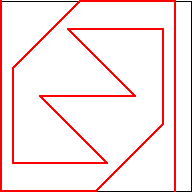s=2, d=45length 15Found by Roger Phillips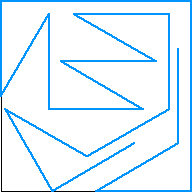s=2, d=30length 20Found by Susan Hoover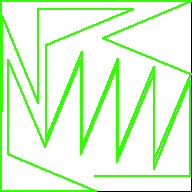s=2, d=22.5length 32Found by Jon K McLean

What are the longest 30o snakes you can find in some small squares? How about 22.5o snakes? What about the longest snakes that fit inside rectangles? circles?

Here are the longest known 45o snakes inside small squares: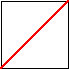s=1/√2, d=45length 1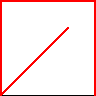s=1, d=45length 4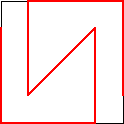s=2-1/√2, d=45length 7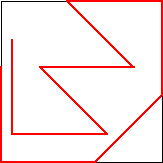s=1+1/√2, d=45length 10s=2, d=45length 15Found by Roger Phillips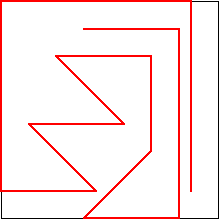s=3-1/√2, d=45length 17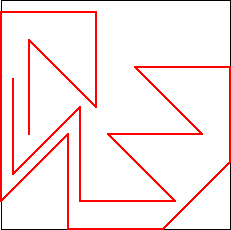s=1+√2, d=45length 19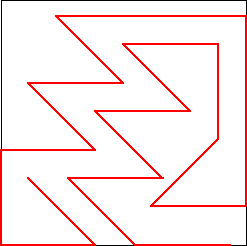s=4-√2, d=45length 21

Here are the longest known 30o snakes inside small squares: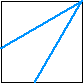s=√3/2, d=30length 2s=1, d=30length 4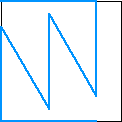s=3-√3, d=30length 7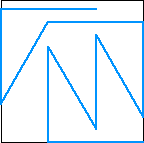s=3/2, d=30length 10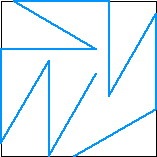s=(5-√3)/2, d=30length 11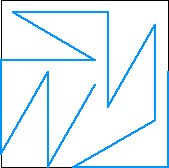s=7/2-√3, d=30length 13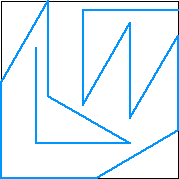s=1+√3/2, d=30length 14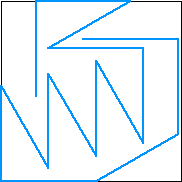s=(9-3√3)/2, d=30length 15

Here are the longest known 22.5o snakes inside small squares: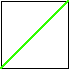s=.708, d=22.5length 1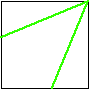s=.924, d=22.5length 2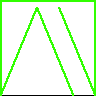s=1, d=22.5length 6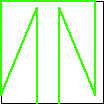s=1.077, d=22.5length 7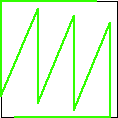s=1.229, d=22.5length 9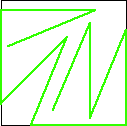s=1.323, d=22.5length 10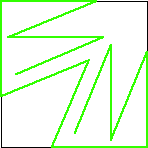s=1.542, d=22.5length 12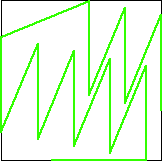s=1.690, d=22.5length 16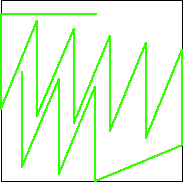s=1.914, d=22.5length 18

Here are the longest known 18o snakes inside small squares: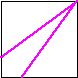s=.810, d=18length 2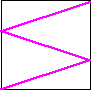s=.952, d=18length 3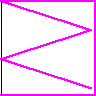s=1, d=18length 6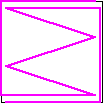s=1.049, d=18length 9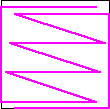s=1.147, d=18length 11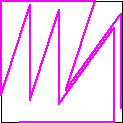s=1.289, d=18length 12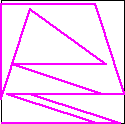s=1.310, d=18length 13

Here are the longest known 45o snake loops inside small squares:s=1, d=45length 4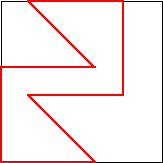s=1+1/√2, d=45length 8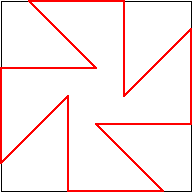s=2, d=45length 12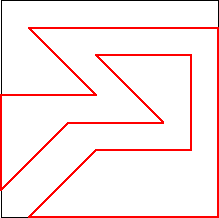s=3-1/√2, d=45length 16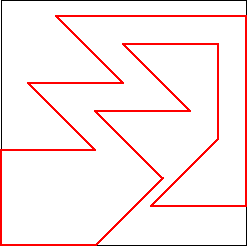s=4-√2, d=45length 18

Here are the longest known 30o snake loops inside small squares:s=1, d=30length 5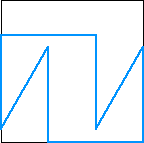s=3/2, d=30length 8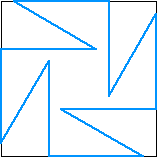s=5/2-√3/2, d=30length 12

Here are the longest 45o snakes inside some small rectangles:

01/√212-1/√21+1/√22√2-1
1/√212-1/√2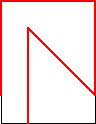1+1/√2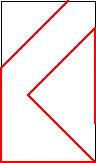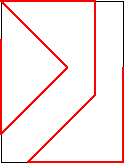2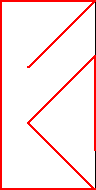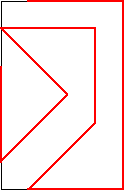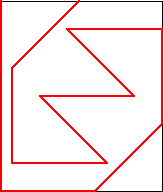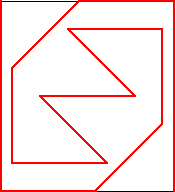Here is the longest 30o snake inside a 2x3 rectangle: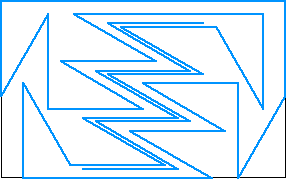length 39(Dave Langers)

Here are the longest 30o snakes inside some equilateral triangles: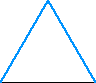s=1, d=30length 2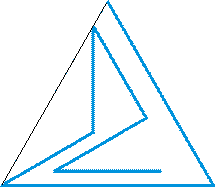s=2, d=30length 9(Serhiy Grabarchuk)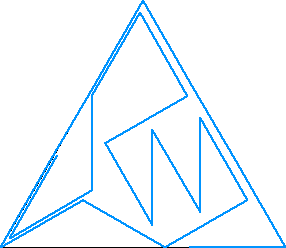s=3, d=30length 21(Dave Langers)

Here are the longest 30o snakes inside some circles: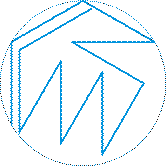r=1, d=30length 12(Peter Grabarchuk)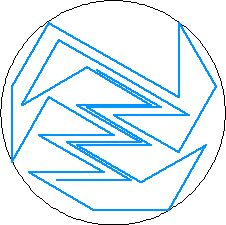r=3/2, d=30length 33(Dave Langers)

Trevor Green and Jeremy Galvagni worked on an efficient snake in rectangles of width 1 and d small. The basic idea is shown below: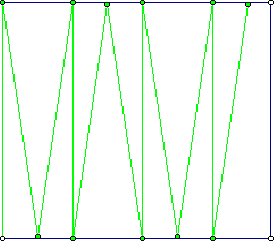Serhiy Grabarchuk found the longest 30o snakes inside regular polygons: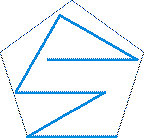d=30length 6d=30length 11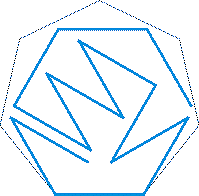d=30length 14d=30length 18

Serhiy Grabarchuk also found the longest 30o snake on the surface of a unit cube. The red lines are on the front faces, and the blue lines are on the back faces.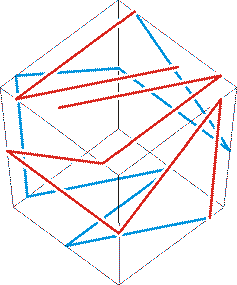In November 2006, Al Zimmerman held a contest to find the largest possible 360/N degree snakes inside a circle of diameter D, for small N and D. Below is a table showing the best results.
Lengths of Longest Snakes
D \ N5789101112
2   7
Andrea
Concaro
8
Andrea
Concaro
11
Andrea
Concaro
9
Andrea
Concaro
11
Andrea
Concaro
12
Peter
Grabarchuk
3 17
Andrea
Concaro
20
Andrea
Concaro
22
Andrea
Concaro
27
Andrea
Concaro
23
Trofimov
34
Trofimov
33
Leonid
Shishlo
4 33
Andrea
Concaro
43
Trofimov
44
Trofimov
59
Trofimov
50
Specht,
Viertel, and
Wohlgemuth
77
Moritz
Franckenstein
71
Hermann
Jurksch
5 57
Trofimov
81
Trofimov
78
Hermann
Jurksch
111
Pfoertner, Sigg,
and Nagel
91
Mark
Beyleveld
148
Hugo
Pfoertner
121
Hermann
Jurksch
6 88
Markus
Sigg
122
Specht,
Viertel, and
Wohlgemuth
123
Michael
van Fondern
192
Specht,
Viertel, and
Wohlgemuth
148
Sigg and
Pfoertner
241
Pfoertner,
Rosenthal,
and Sigg
198
Trofimov
7 125
Jurksch and
Pfoertner
176
Hugo
Pfoertner
179
Markus
Sigg
269
Sigg and
Pfoertner
221
Jurksch and
Pfoertner
369
Sigg and
Pfoertner
288
Hermann
Jurksch
8 172
Hugo
Pfoertner
247
Hermann
Jurksch
248
Hugo
Pfoertner
371
Sigg and
Pfoertner
318
Pfoertner and
Jurksch
9 236
Hugo
Pfoertner
325
Sigg and
Pfoertner
327
Hermann
Jurksch
509
Sigg and
Pfoertner
10 317
Hermann
Jurksch
419
Hermann
Jurksch
444
Pfoertner and
Jurksch
11 393
Hugo
Pfoertner
533
Hermann
Jurksch
12 478
Pfoertner and
Jurksch
13 559
Hermann
Jurksch
14 681
Pfoertner and
Jurksch

If you can extend any of these results, please e-mail me. Click here to go back to Math Magic. Last updated 9/29/20.